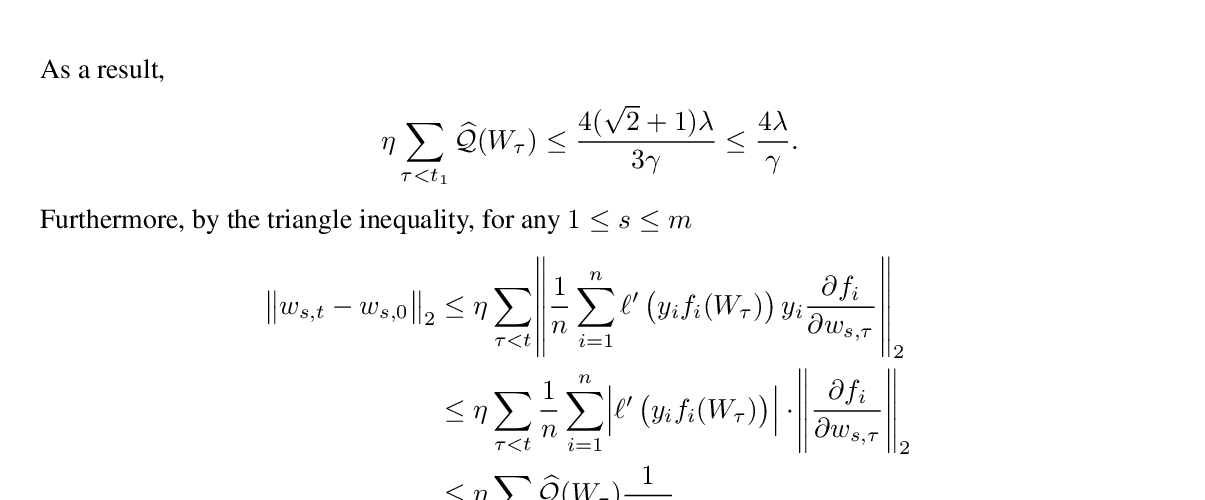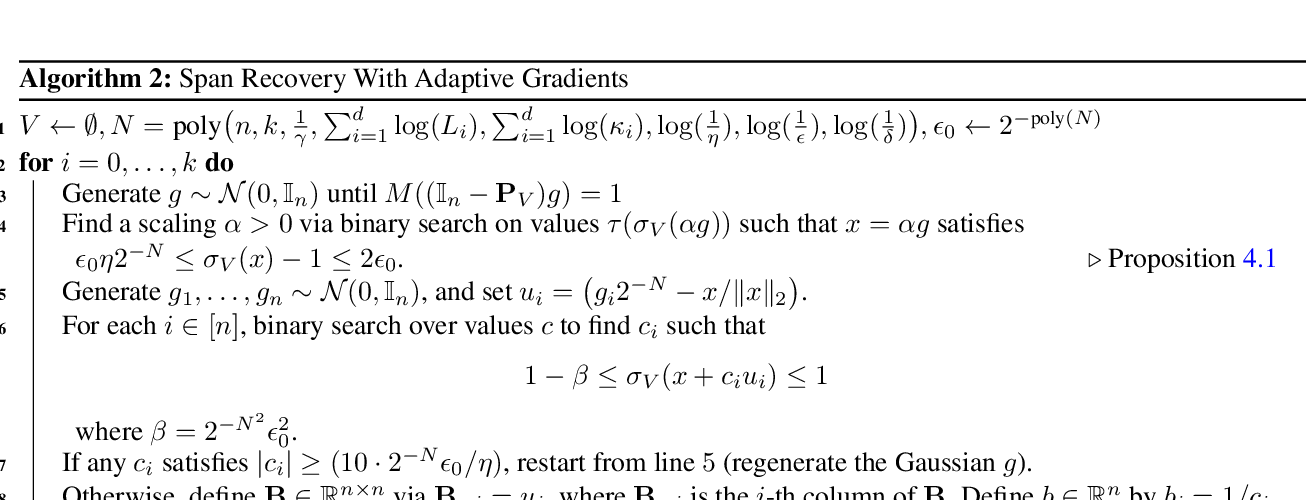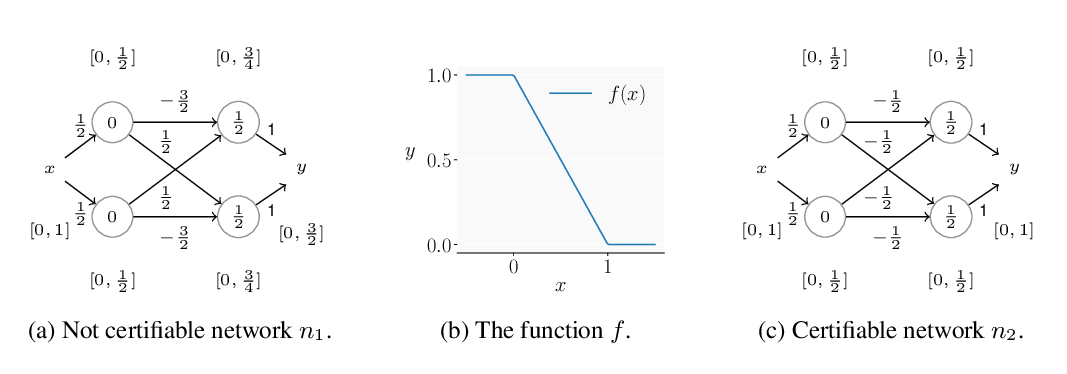## Gradientless Descent: High-Dimensional Zeroth-Order Optimization

### Daniel Golovin, John Karro, Greg Kochanski, Chansoo Lee, Xingyou Song, Qiuyi Zhang

Keywords: optimization, zeroth order optimization

Monday: Optimisation Theory

Abstract: Zeroth-order optimization is the process of minimizing an objective $f(x)$, given oracle access to evaluations at adaptively chosen inputs $x$. In this paper, we present two simple yet powerful GradientLess Descent (GLD) algorithms that do not rely on an underlying gradient estimate and are numerically stable. We analyze our algorithm from a novel geometric perspective and we show that for {\it any monotone transform} of a smooth and strongly convex objective with latent dimension $k \ge n$, we present a novel analysis that shows convergence within an $\epsilon$-ball of the optimum in $O(kQ\log(n)\log(R/\epsilon))$ evaluations, where the input dimension is $n$, $R$ is the diameter of the input space and $Q$ is the condition number. Our rates are the first of its kind to be both 1) poly-logarithmically dependent on dimensionality and 2) invariant under monotone transformations. We further leverage our geometric perspective to show that our analysis is optimal. Both monotone invariance and its ability to utilize a low latent dimensionality are key to the empirical success of our algorithms, as demonstrated on synthetic and MuJoCo benchmarks.

## Similar Papers

##### Polylogarithmic width suffices for gradient descent to achieve arbitrarily small test error with shallow ReLU networks
###### Ziwei Ji, Matus Telgarsky,##### Span Recovery for Deep Neural Networks with Applications to Input Obfuscation
###### Rajesh Jayaram, David P. Woodruff, Qiuyi Zhang,##### Universal Approximation with Certified Networks
###### Maximilian Baader, Matthew Mirman, Martin Vechev,